# Twisting a Surface, or: a Doughnut in Outer Space

Do you know this delicious German pastry?(Photo by Guido Draheim).

It’s called “ Spritzkuchen” or “Spritzring”. I thought of it when I was toying with TikZ and pgfplots. It’s similar to a twisted torus. Today I dealt with it again, when writing on the French TeX question and answer site TeXnique.fr, with the help of my French TeX friends Patrick Bideault and Denis Bitouzé.

Before I come to code and explanation, here are some of the steps and images in the construction process, using pgfplots.

Let’s get a representative cross section. In polar coordinates, the sine function sin(x) gives a circle, sin(3x) are three leaves, we add a bit radius (1.25) as piece in the middle. This gives us the function sin(3x) + 1.25 in polar coordinates: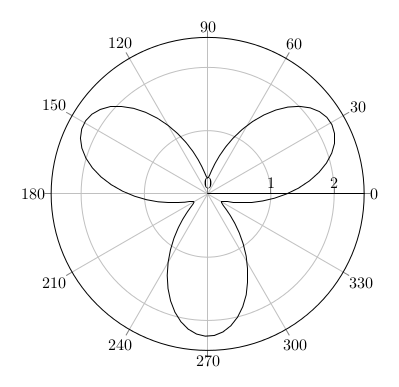We embed it in the space, such as in the xy plane with z=0 as (x,y,z)(t) = ( cos(t)(sin(3t)+1.25), sin(t)(sin(3t) + 1.25), 0 ):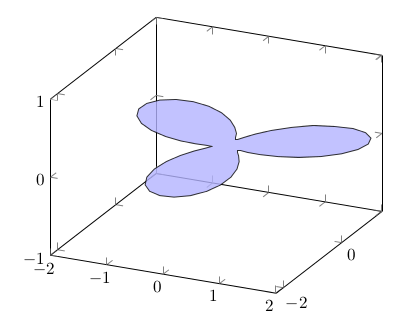Or differently turned: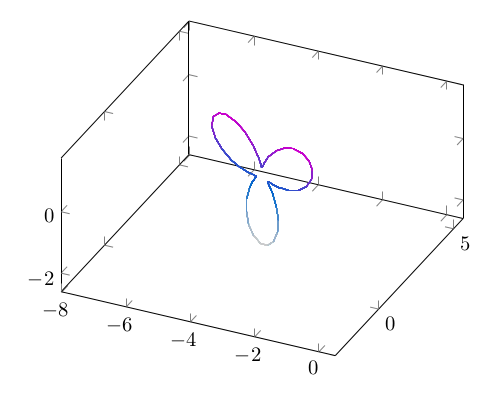We can move it straight through the space for example by drawing in the xz plane and let y run linearly: (x,y,z)(t) = ( cos(t)(sin(3t)+1.25), t, sin(t)(sin(3t)+1.25) ). In space it looks this way: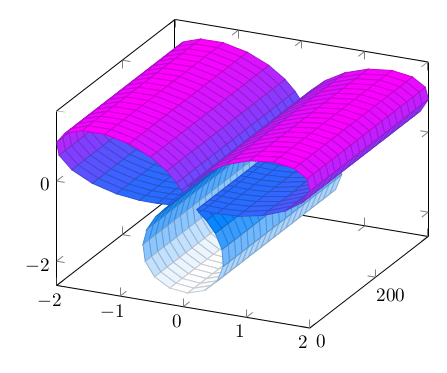But we want to turn it. Moving it in a circle in space, we use a torus map, such as:

x(t,s) = (2+cos(t))cos(s+pi/2)
y(t,s) = (2+cos(t))sin(s+pi/2)
z(t,s) = sin(t)

We connect it with our original function:

x(t,s) = (6+(sin(3t)+1.25)cos(t))cos(s)
y(t,s) = (6+(sin(3t)+1.25)cos(t))sin(s)
z(t,s) = (sin(3t)+1.25)sin(t)

Here is a half section, moved half a turn: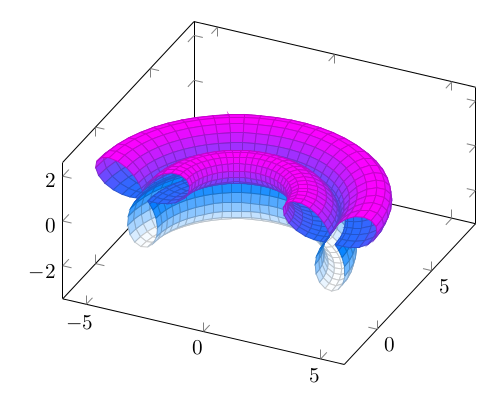And fully rotated: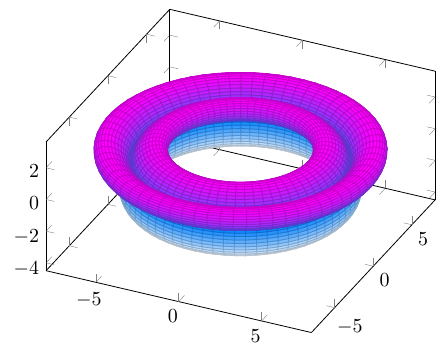And now to the twist. We can twist it by adding a multiple oft t resp. y to the argument of the function. So we achieve a rotation with growing y value: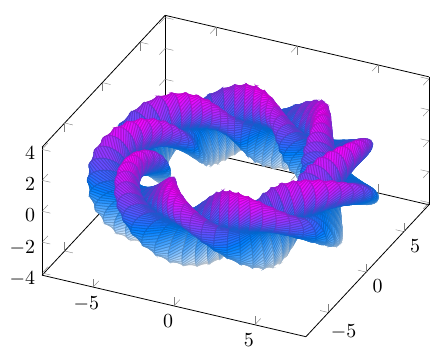Not that we did the math and the TeX, let’s frame it. Music: from the Soundtrack of “Independence Day”, as today is the 4th of July and I hang around in the US, in Miami, Florida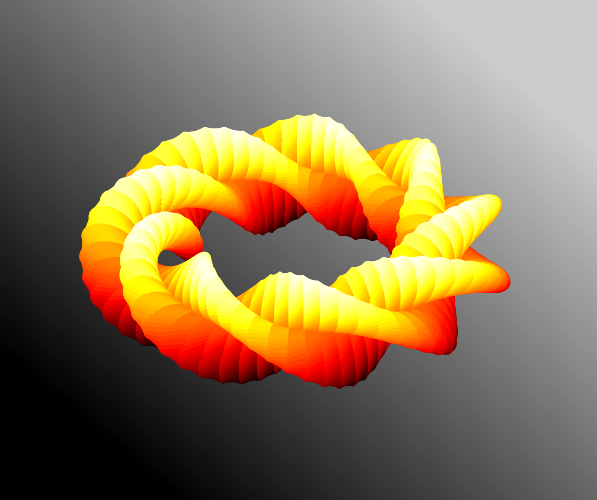Ok, code or it didn’t happen.

% Compile with LuaLaTeX because of heavy computations, too hard for pdfLaTeX
\RequirePackage{luatex85}% Only as long as the standalone class is not yet compatible to the newest LuaTeX
\documentclass{standalone}
\usepackage{pgfplots}
\usetikzlibrary{backgrounds}
\begin{document}
\begin{tikzpicture}
\begin{axis}[axis equal,
hide axis,
/tikz/background rectangle/.style = {
left color    = black,
right color   = black!20,
},
show background rectangle

]
surf,
miter limit = 1,
domain      = 0:360,
y domain    = 0:360,
samples     = 100,
samples y   = 70,
z buffer    = sort,
colormap/hot2,

]
( {(6+(sin(3*(x+3*y))+1.25)*cos(x))*cos(y)},
{(6+(sin(3*(x+3*y))+1.25)*cos(x))*sin(y)},
{((sin(3*(x+3*y))+1.25)*sin(x))} );
\end{axis}
\end{tikzpicture}
\end{document
}

All the code and the explanation can be read here – it developed over time:

## 2 thoughts

1.Jörg says:

Nice, but I can’t get it to work:

! Package PGF Math Error: Unknown function `em’ (in ‘ {(6+(sin(3(x+3y)
)+1.25)cos(x))cos(y)}’).

I am using TexLive 2017

1.stefan says:

Hi Jörg,

thanks! The Markdown plugin affected the code example. It makes *text* to emphasized/italic text, according to Markdown syntax, even in a code box, so we got the em HTML tags. That should not happen. I fixed it.

Stefan